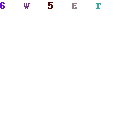The EAN-13 barcode format is the standard barcode used on everyday products across the world, except in the US, where the UPC-A barcode is more popular.EAN-13 Barcode

The EAN-13 barcode is a 13 digit barcode, where the first two or three digies are the country code where the manufacturer of the product is registered. The next nine or ten digits are data digits, which adds up to 12 digits. The last digit is the checksum digit.

To calculate the checksum (also known as the parity), we first need add up the 12 digits of the barcode with the weighting applied. For the EAN-13 barcode, the weighting of each digit which is in an odd-numbered position is 1 and for even-numbered positions, the weighting is 3. To find the sum, we need to multiply each digit by its weighting before adding it together.

Once we have the weighted sum, we apply a modulo 10 to the weighted sum, which gets the remainder after applying a modulo of 10 to the weighted sum.

The parity is then 10 – (modulo 10 of the weighted sum).

Now that we have a 13 digit number, we can encode the data we need to print out a barcode. Each digit is represented by a series of bars and spaces. I will represent a bar as 1 and a space as 0 for the rest of the tutorial.

EAN-13 splits up the 12 digits we need to print into two blocks of 6 with a guard bar at the beginning and end of the barcode and one in the middle of the barcode too. The end guard bars are encoded as 101, while the middle guard bar is 01010.

The parity of the barcode is not printed directly, but is rather used to determine which set of encodings we are going to use for each character, which complicates the encoding of the barcode slightly.

For first 6 digits (the left hand side of the barcode) there is an odd and even encoding. The parity defines which encoding to use. The parity check has a 6 digit binary string, where each digit determines whether to use the odd or even encoding set at the position in the parity string. So for example, for a parity of 3, the binary string is 110001, which means that at position 2, we need to use the odd set, and at position 3 we need to use the even set, and so on.

The parity encodings are as follows:

 0 111111 1 110100 2 110010 3 110001 4 101100 5 100110 6 100011 7 101010 8 101001 9 100101

For the left hand side encodings then, the encodings are as follows:

 Digit Odd Even 0 0001101 0100111 1 0011001 0110011 2 0010011 0011011 3 0111101 0100001 4 0100011 0011101 5 0110001 0111001 6 0101111 0000101 7 0111011 0010001 8 0110111 0001001 9 0001011 0010111

The right hand side encodings (the last 6 digits) do not need this and are just encode straight.
The encodings are as follows:

 0 1110010 1 1100110 2 1101100 3 1000010 4 1011100 5 1001110 6 1010000 7 1000100 8 1001000 9 1110100

Here is the code to encode the barcode. The full source code is available here

```namespace BarcoderLib
{
public class BarcodeEAN13
{
private string gLeftGuard = "101";
private string gCentreGuard = "01010";
private string gRightGuard = "101";
private string[] gLHOdd = { "0001101", "0011001", "0010011", "0111101", "0100011", "0110001", "0101111", "0111011", "0110111", "0001011"};
private string[] gLHEven = { "0100111", "0110011", "0011011", "0100001", "0011101", "0111001", "0000101", "0010001", "0001001", "0010111"};
private string[] gRH = {"1110010", "1100110", "1101100", "1000010", "1011100", "1001110", "1010000", "1000100", "1001000", "1110100"};
private string[] gParity = {"111111", "110100", "110010", "110001", "101100", "100110", "100011", "101010", "101001", "100101" };
private int[] gWeighting = { 1, 3, 1, 3, 1, 3, 1, 3, 1, 3, 1, 3 };
private string gLongBars = "11100000000000000000000000000000000000000000011111000000000000000000000000000000000000000000111";

public Bitmap Encode(string message)
{
string encodedMessage;
string fullMessage;

Bitmap barcodeImage = new Bitmap(250, 100);
Graphics g = Graphics.FromImage(barcodeImage);

Validate(message);
fullMessage = message + CalcParity(message).ToString().Trim();
encodedMessage = EncodeBarcode(fullMessage);

PrintBarcode(g, encodedMessage, fullMessage, 250, 100);

return barcodeImage;
}
private void Validate(string message)
{

Regex reNum = new Regex(@"^\d+\$");
if (reNum.Match(message).Success == false)
{
throw new Exception("Encode string must be numeric");
}

if (message.Length != 12)
{
throw new Exception("Encode string must be 12 digits long");
}
}

private void PrintBarcode(Graphics g, string encodedMessage, string message, int width, int height)
{
SolidBrush whiteBrush = new SolidBrush(Color.White);
SolidBrush blackBrush = new SolidBrush(Color.Black);
Font textFont = new Font(FontFamily.GenericMonospace, 9, FontStyle.Regular);
g.FillRectangle(whiteBrush, 0, 0, width, height);

int xPos = 20;
int yTop = 10;
int barHeight = 50;
int barGuardHeight = 7;

for (int i = 0; i < encodedMessage.Length; i++)
{
if (encodedMessage[i] == '1')
{
if (gLongBars[i] == '1')
{
g.FillRectangle(blackBrush, xPos, yTop, 1, barHeight + barGuardHeight);
}
else
{
g.FillRectangle(blackBrush, xPos, yTop, 1, barHeight);
}
}
xPos += 1;
}

xPos = 20;
yTop += barHeight - 3;
g.DrawString(message.ToString().Trim(), textFont, blackBrush, xPos - 14, yTop);

xPos += 2;
for (int i = 1; i < 7; i++)
{
g.DrawString(message[i].ToString().Trim(), textFont, blackBrush, xPos, yTop);
xPos += 7;
}
xPos += 3;

for (int i = 7; i < message.Length; i++)
{
g.DrawString(message[i].ToString().Trim(), textFont, blackBrush, xPos, yTop);
xPos += 7;
}

}

private string EncodeBarcode(string message)
{
int i;
char parity;

string encodedString = gLeftGuard;
int parityCode = Convert.ToInt32(message.ToString());

for (i = 1; i < 7; i++)
{
parity = gParity[parityCode][i-1];
if (parity == '1')
{
encodedString += gLHOdd[Convert.ToInt32(message[i].ToString())];
}
else
{
encodedString += gLHEven[Convert.ToInt32(message[i].ToString())];
}
}
encodedString += gCentreGuard;

for(i = 7; i < 13; i++)
{
encodedString += gRH[Convert.ToInt32(message[i].ToString())];
}
encodedString += gRightGuard;

return encodedString;
}

private int CalcParity(string message)
{
int sum = 0;
int parity = 0;

for(int i = 0; i < 12; i++)
{
sum += Convert.ToInt32(message[i].ToString()) * gWeighting[i];
}

parity = 10 - (sum % 10);
if (parity == 10)
{
parity = 0;
}
return parity;

}

}
}
```